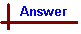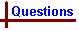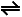More Acids and Bases 2 Link to Data Page Your feedback on these self-help problems is appreciated. Click here to send an e-mail. For on-line help on this topic, please see the following Chemcal modules: Acids and Bases (strong acids and bases; conjugate acid/base pairs; pH;self-dissociation of water) Weak Acids and Bases (weak acids; weak bases; Ka and Kb) Calculations with Weak Acids and Bases (calculations involving weak acids and bases and buffers) Acid-base Titrations (titration curves for acid/base titrations; indicators) Shortcut to Questions Q: 1 2 3 4 5 6 7 8 1 Suggest conjugate acid/base pairs to give the following buffered pH values: (1.) 3.0 (2.) 5.0 (3.) 7.0 (4.) 9.0 (5.) 10.3 (6.) 13.02 What buffered pH would be obtained from solutions of equimolar amounts of the following conjugate acid/base pairs: (1.) HPO42- / PO43- (2.) H3BO3 / H2BO3- (3.) HF / F- (4.) HS- / S2- (5.) ClCH2COOH / ClCH2CO2-3 What is the pH of 1.00 M ammonia solution (100 cm3) in which ammonium chloride (9.30 g) has been dissolved?4 (1.) What is the molarity of a water-solution of propionic acid of pH 3.00? (2.) What mass of potassium propionate must be added to one litre of this solution to change the pH to 5.00?5 What volume of 10.0 M hydrochloric acid must be added to 0.100 M sodium lactate (1.00litre) to give a solution with pH = 3.86?6 (1.) The major buffering action in blood plasma is due to the hydrogencarbonate ion / carbonic acid system. The hydrogencarbonate ion concentration in a sample of blood plasma was found to be 0.0230 M. Calculate the concentration of carbonic acid in this sample if its pH is 7.40 at 37 ° C. (pKA H2CO3 at 37 ° C = 6.10) (2.) To this blood plasma (100 cm3) is added a 0.056 M solution of sodium hydroxide (0.20 cm3). What is the new pH of the plasma?7 Calculate the pH at the equivalence point for the following acid/base titrations with 0.20 M solutions and choose a suitable indicator for each titration. (1.) NH3 with HCl (2.) NaOH with HCN (3.) KOH with HOCl (4.) KOH with HNO3 (5.) NH2OH with HCl (6.) NaOH with HNO28 A 0.10 M solution of an acid / base indicator has a pH of 5.00. Would this indicator be suitable for the titration of a weak acid with a strong base or a weak base with a strong acid? Give a clear reason for your answer.More Acids and Bases 2 (Answers) 1 A buffer is most effective when [weak acid] = [conjugate base]. Under this condition, pH of the solution = pKA of the weak acid. To select a buffer, choose a weak acid for which pKA ~ pH desired, and fine tune the buffer to give the precise pH required by adjusting the acid / base ratio. From the list of acid pKA values, the following acid / base pairs are suitable: (1.) pH = 3.0 HF has a pKA of 3.17, so a HF / F- pair would be suitable. As the required pH is on the acidic side of pKA(HF), an excess of HF would be required. (2.) pH = 5.0 CH3COOH has a pKA of 4.76, so a CH3CHOOH / CH3CHOO- pair would be suitable. As the required pH is on the basic side of pKA(CH3COOH), an excess of CH3COO- would be required. (3.) pH = 7.0 H2PO4- has a pKA of 7.20, so a H2PO4- / HPO42- pair would be suitable. As the required pH is on the acidic side of pKA(H2PO4-), an excess of H2PO4- would be required. (4.) pH = 9.0 NH4+ has a pKA of 9.24, so a NH4+ / NH3 pair would be suitable. As the required pH is on the basic side of pKA(NH4+), an excess of NH3 would be required. (5.) pH = 10.3 HCO3- has a pKA of 10.33, so a HCO3- / CO32- pair would be suitable. As the required pH is on the acidic side of pKA(HCO3-), an excess of HCO3- would be required. (6.) pH = 13.0 HS- has a pKA of 12.89, so a HS- / S2- pair would be suitable. As the required pH is on the basic side of pKA(HS-), an excess of S2- would be required.2 KA = [H3O+] [A-] / [HA] Since equimolar amounts of weak acid and its conjugate base are present in each of the buffer solutions, [A-] / [HA] = 1 and thus KA = [H3O+]. Therefore pH = pKA of the weak acid in each conjugate acid/base pair. So, from the data sheet: (1.) pH = pKA = 12.36 (2.) pH = pKA = 9.24 (3.) pH = pKA = 3.17 (4.) pH = pKA = 12.89 (5.) pH = pKA = 2.863 Volume of solution is 100 mL. [NH3] = 1.00 mol L-1 Moles of NH4Cl = 9.30 g / 53.49 g mol-1 = 0.174 mol [NH4+] = 0.174 mol / 0.100 L = 1.74 mol L-1 pKA(NH4+) = 9.24 KA(NH4+) = 10-9.24 KA = [H3O+] [A-] / [HA] = [H3O+][NH3] / [NH4+] [H3O+] = KA [NH4+] / [NH3] = 10-9.24 x 1.74 / 1.00 = 1.00 x 10-9 M pH = -log [H3O+] = -log 1.00 x 10-9 mol L-1 = 9.004 (1.) H2O + CH3CH2COOHH3O+ + CH3CH2CO2- pKA (CH3CH2COOH) = 4.87 KA (CH3CH2COOH) = 10-4.87 KA = [CH3CH2CO2-] [H3O+] / [CH3CH2COOH] = [H3O+]2 / [CH3CH2COOH] [H3O+] for a pH of 3.00 = 0.0010 mol L-1 ∴ [CH3CH2COOH] = (0.0010 )2 / 1.35 x 10-5 = 0.074 M (2.) KA = [H3O+] [A-] / [HA] Now the desired pH is 5.00, so [H3O+] = 1.00 x 10-5 M ∴ [CH3CH2CO2-] = KA [CH3CH2COOH] / [H3O+] = 10-4.87 x 0.074 / 1.00 x 10-5 = 0.10 M The volume of the solution is one litre, so 0.10 mol CH3CH2CO2K is required. Mass CH3CH2CO2K = moles x molar mass = 0.10 mol x 112.14 g mol-1 = 11 g5 pKA lactic acid = 3.86. Note that lactic acid is a monoprotic acid. If pH is equal to pKA, it follows that [HA] = [A-] [A-] = [lactate] = 0.100 mol L-1 Lactic acid is a weak acid, so H3O+ will react with sodium lactate to give lactic acid. There is 0.100 mole of lactate ion present, so to obtain equimolar amounts of lactate ion and lactic acid, we need to add enough H3O+ to react with half the lactate ion present. This will provide us with a solution containing 0.0500 mole of lactate and 0.0500 mole of lactic acid. To produce 0.0500 mole of lactic acid we need 0.0500 mole of H3O+, which is obtained from 0.0500 mole of hydrochloric acid (since both lactic acid and hydrochloric acid are monoprotic). ∴ Volume 10.0 M hydrochloric acid required = moles hydrochloric acid / molarity of hydrochloric acid = 0.0500 mol / 10.0 mol L-1 = 0.00500 L = 5.00 mL.6 The acid / base pair in this system is H2CO3 / HCO3-. (1.) [HCO3-] = 0.0230 mol L-1 pH = 7.40 ∴ [H3O+] = 10-7.40 pKA (H2CO3) = 6.10 (at 37 ° C) ∴ KA (H2CO3) = 10-6.10 KA = [H3O+] [HCO3-] / [H2CO3] [H2CO3] = [H3O+] [HCO3-] / KA = 10-7.40 x 0.0230 / 10-6.10 M = 1.2 x 10-3 M (2.) The buffer system reacts to the strong base OH- by consuming H2CO3 and producing HCO3- : OH- + H2CO3 → HCO3- + H2O Assuming that all OH- is converted to HCO3-, we can calculate the new equilibrium amounts after addition of NaOH: Initial [HCO3-] = 2.30 x 10-2 mol L-1 Initial [H2CO3] = 1.2 x 10-3 mol L-1 Volume of solution = 0.100 L, so in this volume initial moles H2CO3 = volume x molarity = 1.2 x 10-4 mol and initial moles HCO3- = 2.30 x 10-3 mol Moles of hydroxide ion added = volume x molarity = 0.20 x 10-3 x 0.056 mol = 1.12 x 10-5 mol. Addition of the 1.12 x 10-5 mole of NaOH leads to new amounts of the buffer pair forming in response to the strong base OH -. The result at equilibrium is an increase in the moles of HCO3- and an equal decrease in the moles of H2CO3. Eqb. moles HCO3- = 2.30 x 10-3 mol + 1.12 x 10-5 mol = 2.31 x 10-3 mol. Eqb. moles H2CO3 = 1.2 x 10-4 mol - 1.12 x 10-5 mol = 1.09 x 10-4 mol. Volume = 0.100 L ∴ Eqb. [HCO3-] = 2.3 x 10-2 M ∴ Eqb. [H2CO3] = 1.2 x 10-3 M1 Calculating pH with these new amounts of acid and base: KA = [H3O+] [HCO3-] / [H2CO3] [H3O+] = KA [H2CO3] / [HCO3-] ∴ [H3O+] = KA x [H2CO3] / [HCO3-] = (10-6.10 x 1.09 x 10-3) / (2.31 x 10-2) = 3.75 x 10-8 M ∴ pH = -log (3.75 x 10-8) = 7.437 The equivalence point is reached when stoichiometric amounts of acid and base have reacted. (1.) NH3 + H3O+ → NH4+ + H2O There is a change in volume during titration. At the equivalence point equal volumes of 0.20 M HCl and 0.20 M NH3 have reacted because the NH3 and hydrochloric acid solutions are of the same molarity. This leads to a solution which is 0.10 M in NH4+ - not 0.20 M! This is the concentration used to calculate pH below. At the equivalence point the solution is 0.10 M in NH4+ and Cl-. Finding the pH at the equivalence point is the same as finding the pH of a 0.10 M NH4Cl solution. Cl- is the conjugate base of a strong acid, HCl, so it does not hydrolyse water and plays no role in altering pH. The ammonium ion however is a weak acid and thus does alter pH: NH4+ + H2ONH3 + H3O+ Finding [H3O+]: pKA(NH4+) = 9.24 ∴ KA(NH4+) = 10-9.24 Using KA = [H3O+] [A-] / [HA] : KA(NH4+) = [H3O+] [NH3] / [NH4+] From the stoichiometry of the equation for the dissociation of ammonium ion given above, [NH3] = [H3O+]. Therefore KA(NH4+) = [H3O+]2 / [NH4+] ∴ [H3O+] = (KA(NH3) x [NH4+])½ = (5.75 x 10-10 x 0.10)½ = 7.58 x 10-6 M. pH = -log [H3O+] = -log (7.58 x 10-6) = 5.12. A suitable indicator will have a pKA about the same as the pH at the titration equivalence point. Referring to a table of indicators and their pKA values, a suitable indicator for this reaction would be methyl red, which has a pKA in the vicinity of 5.12 (2.) OH- + HCN → CN- + H2O Mixing 0.20 M solutions of NaOH and HCN leads to a solution which is 0.10 M in both Na+ and CN-. The sodium ions present do not affect the pH, and are not considered further. The pH of 0.10 M CN- solution, a weak base, is found starting with the pKA of its weak conjugate acid, HCN. ∴ KA(HCN) = 10-9.22 The following equilibrium will be considered: CN- + H2OHCN + OH- KB(CN-) = KW / KA(HCN) = 1.0 x 10-14 / (6.03 x 10-10) = 1.66 x 10-5 KB(CN-) = [HCN] [OH-] / [CN-] From the stoichiometry of the reaction above, [HCN] = [OH-] : KB(CN-) = [OH-]2 / [CN-] ∴ [OH-] = (KB(CN-) x [CN-])½ = (1.66 x 10-5 x 0.10 )½ = 1.29 x 10-3 M. [H3O+] = KW / [OH-] = 1.00 x 10-14 / (1.29 x 10-3) = 7.75 x 10-12 M pH = -log [H3O+] = -log (7.75 x 10-12) = 11.11. A suitable indicator would be alizarin yellow, which has a pKA in the vicinity of 11.11 (3.) Reaction products are K+ and OCl-. Only the OCl- enters into hydrolysis reaction with water to form HOCl and OH- in equal quantities as shown in the following equation: H2O + OCl-HOCl + OH- i.e. OCl- ion is a weak base. Using the same method as in (2) above, pH = 10.27, phenolphthalein indicator (4.) Reaction products are K+ and NO3-. Neither of these undergo acid / base reactions with the solvent, water, so pH = 7.00, bromothymol blue indicator (5.) Reaction products are NH3OH+ and Cl-. The chloride ion, being the conjugate of a strong acid, does not enter into a hydrolysis reaction with water but the NH3OH+ ion is a weak acid which reacts slightly with water according to the equation NH3OH+ + H2ONH2OH + H3O+ Using the same method as in (1) above, pH = 3.48 and methyl yellow or methyl orange indicator would be suitable. (6.) Reaction products are Na+ and NO2-. Only the NO2- enters into hydrolysis reaction with water to form HNO2 and OH- in equal quantities as shown in the following equation: H2O + NO2-HNO2 + OH- i.e. NO2- ion is a weak base. Using the same method as in (2) above, pH = 8.07, cresol red indicator.8 The indicator, represented as HIn, is a weak acid. As the titration proceeds, the acid form of the indicator, HIn, is converted to its conjugate base, In-, and water. The colour change of any indicator occurs when [HIn] in the solution = [In-], ie when pH of the solution = pKA of the indicator. From the information given, pKA can be determined. HIn+ H2OIn- + H3O+ KA(HIn) = [H3O+] [In-] / [HIn] pH of 0.10 M indicator solution = 5.00, ∴ [H3O+] = 1.0 x 10-5 M [In-] = [H3O+], and we assume [HIn]init = [HIn]eqb: KA(HIn) = [H3O+]2 / [HIn]init = (1.0 x 10-5)2 / 0.10 = 1.0 x 10-9 ∴ pKA(HIn) = -log KA = 9.00. ∴ The indicator undergoes colour change at pH = 9.00. This would be suitable for the titration of a weak acid with a strong base.